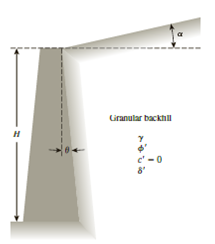Chapter 14, Problem 14.10PPrinciples of Geotechnical Enginee...

9th Edition
Braja M. Das + 1 other
ISBN: 9781305970939

Solutions

Chapter
SectionPrinciples of Geotechnical Enginee...

9th Edition
Braja M. Das + 1 other
ISBN: 9781305970939
Textbook Problem

Redo Problem 14.8 when θ = 0° and α = 12° (Figure 14.7 and Table 14.6).14.8 Refer to Figure 14.19. Given: θ = 0, α = 0°, H = 5 m, γ = 15 kN/m3, ϕ ′ = 30 ° , and δ ′ = 15 ° . Estimate the passive force, Pp, per unit length of the wall using Caquot and Kerisel’s solution (Figure 14.6 and Table 14.6).Figure 14.19

To determine

Find the passive force Pp per unit length of the wall using Caquot and Kerisel’s solution.

Explanation

Given information:

The height (H) of the retaining wall is 5.0 m.

The soil friction angle ϕ is 30°.

The angle of wall friction δ is 15°.

The given angle θ value is 0°.

The given angle α value is 12°.

The unit weight γ of the granular backfill is 15kN/m3.

Calculation:

Calculate the passive force Pp per unit length of the wall using the Caquot and Kerisel’s solution.

Pp=12γH12Kp (1)

Here, Kp is the passive earth pressure coefficient.

Refer Figure 14.7 “Caquot and Kerisel’s solution for Kp” in the text book.

Determine the ratio of angle of wall friction to the soil friction angle.

δϕ=15°30°=0.50

Take the passive earth pressure coefficient Kp for the soil having friction angle of 30° and ratio of angle of wall friction to the soil friction angle 0

Still sussing out bartleby?

Check out a sample textbook solution.

See a sample solution

The Solution to Your Study Problems

Bartleby provides explanations to thousands of textbook problems written by our experts, many with advanced degrees!

Get Started

In your own words, explain the water cycle.

Engineering Fundamentals: An Introduction to Engineering (MindTap Course List)

What is privacy in an information security context?

Principles of Information Security (MindTap Course List)

What is the maximum width of the cover pass? Why?

Welding: Principles and Applications (MindTap Course List)